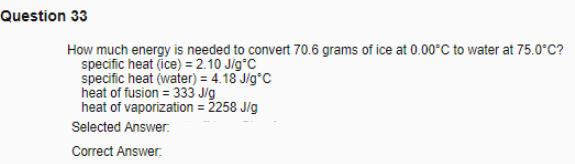# Problem: How much energy is needed to convert 70.6 grams of ice at 0.00°C to water at 75.0°C? specific heat (ice) = 2.10 J/g°C specific heat (water) = 4.18 J/g°C heat of fusion = 333 J/g heat of vaporization = 2258 J/g

🤓 Based on our data, we think this question is relevant for Professor Smith's class at UH.

###### FREE Expert Solution###### Problem Details

How much energy is needed to convert 70.6 grams of ice at 0.00°C to water at 75.0°C?

specific heat (ice) = 2.10 J/g°C

specific heat (water) = 4.18 J/g°C

heat of fusion = 333 J/g

heat of vaporization = 2258 J/g